Describing motion along a line

Start this free course now. Just create an account and sign in. Enrol and complete the course for a free statement of participation or digital badge if available.

Free course

# 5 Uniformly accelerated motion along a line

## 5.1 Describing uniformly accelerated motion

An important special case of non-uniform motion along a line is that which arises when an object is subjected to constant acceleration. This kind of motion is called uniformly accelerated motion. An object falling under gravity near to the surface of the Earth, such as the apple of Figure 24, provides an approximate realisation of such motion. (Air resistance, which increases with speed, prevents the acceleration from being truly constant in such cases.) A more precise realisation of uniformly accelerated motion is provided by an object falling under gravity close to the surface of an airless body such as the Moon (see Figure 38) or by a falling object in an evacuated (i.e. airless) drop-tower or drop-shaft of the kind discussed in Section 1 of this course.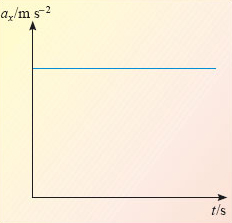Figure 38: The acceleration-time graph for an object with constant (positive) acceleration

The acceleration-time graph for a uniformly accelerated body is simple; it's just a horizontal line of the kind shown in Figure 38. The value of this constant acceleration (which may be positive or negative) represents the gradient of the velocity-time graph at any moment. It follows that the velocity-time graph must have the same gradient at all points and must therefore be a straight line of the kind shown in Figure 39. Note that the sign of the acceleration determines whether the velocity-time graph slopes up or down, and the value of the acceleration determines the precise value of the gradient. However, the acceleration does not determine the initial value of v﻿x so we have arbitrarily chosen a point on the v﻿x -axis to represent this value and labelled it u﻿x. From Figure 38, we deduce that if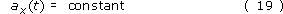then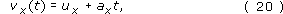where a﻿x represents the constant value of a﻿x(t).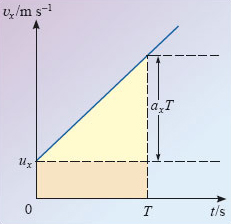Figure 39: A velocity-time graph that is consistent with the acceleration-time graph of Figure 38. Note that the intercept has been chosen arbitrarily; only the gradient is determined by the acceleration

It's rather more difficult to deduce the position-time graph that corresponds to uniformly accelerated motion, but the steady change of velocity with time certainly implies that the gradient of the position-time graph must also change steadily with time. In fact, given a velocity-time graph like that in Figure 39, the corresponding position-time graph will be of the general form shown in Figure 40.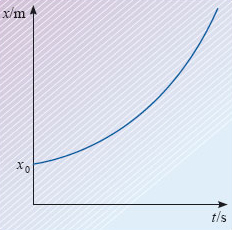Figure 40: A position-time graph that is consistent with the acceleration-time graph of Figure 38 and the velocity-time graph of Figure 39. Note that the intercept has been chosen arbitrarily; only the gradient is determined by velocity

Once again, the intercept with the vertical axis (representing the initial position at t = 0) is not determined by anything we have said so far; it has therefore been chosen arbitrarily and labelled x0. The curve however is not arbitrary since the displacement from x0 at any particular time t = T is determined by the area under the velocity-time graph between t = 0 and t = T. That area will be the sum of two parts, a rectangle of height u﻿x and base length T, and a triangle of height a﻿x﻿T and base length T. Since the area of a triangle is half the product of its height and its base length, it follows that the displacement from x﻿0 at time T will be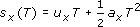and the position of the uniformly accelerating particle, at any time t, will be x﻿(﻿t﻿) = x﻿0 + s﻿x﻿(﻿t﻿), that is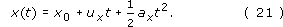Equations 19, 20 and 21 provide an essentially complete description of uniformly accelerated motion in one dimension and have many applications. They are not the most common form of the equations of uniform acceleration. We will discuss those in the next subsection, but before doing so let's use the method of differentiation to confirm the consistency of the equations we have deduced.

Starting from Equation 21, the right-hand side of which is a quadratic function of t, we expect to find that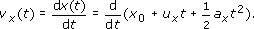Using the rules and results of Table 6 to carry out the differentiation (which is just like that in Question 18) we find that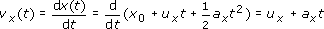in complete agreement with Equation 20. Similarly, differentiating the linear function that appears on the right-hand side of Equation 20, we expect to find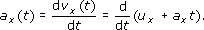Again, performing the differentiation, using Table 6, confirms our expectations: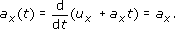We see that in this case, ax(t) is just the constant acceleration ax from which we started.

This short exercise in checking consistency gives just a hint of the immense power of differentiation to simplify a wide range of tasks and investigations.

Returning now to the description of uniformly accelerated motion, let's gather together our results so far: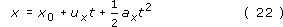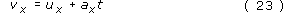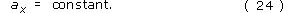These are the equations we shall use, rearrange and extend in the next subsection.

S207_2